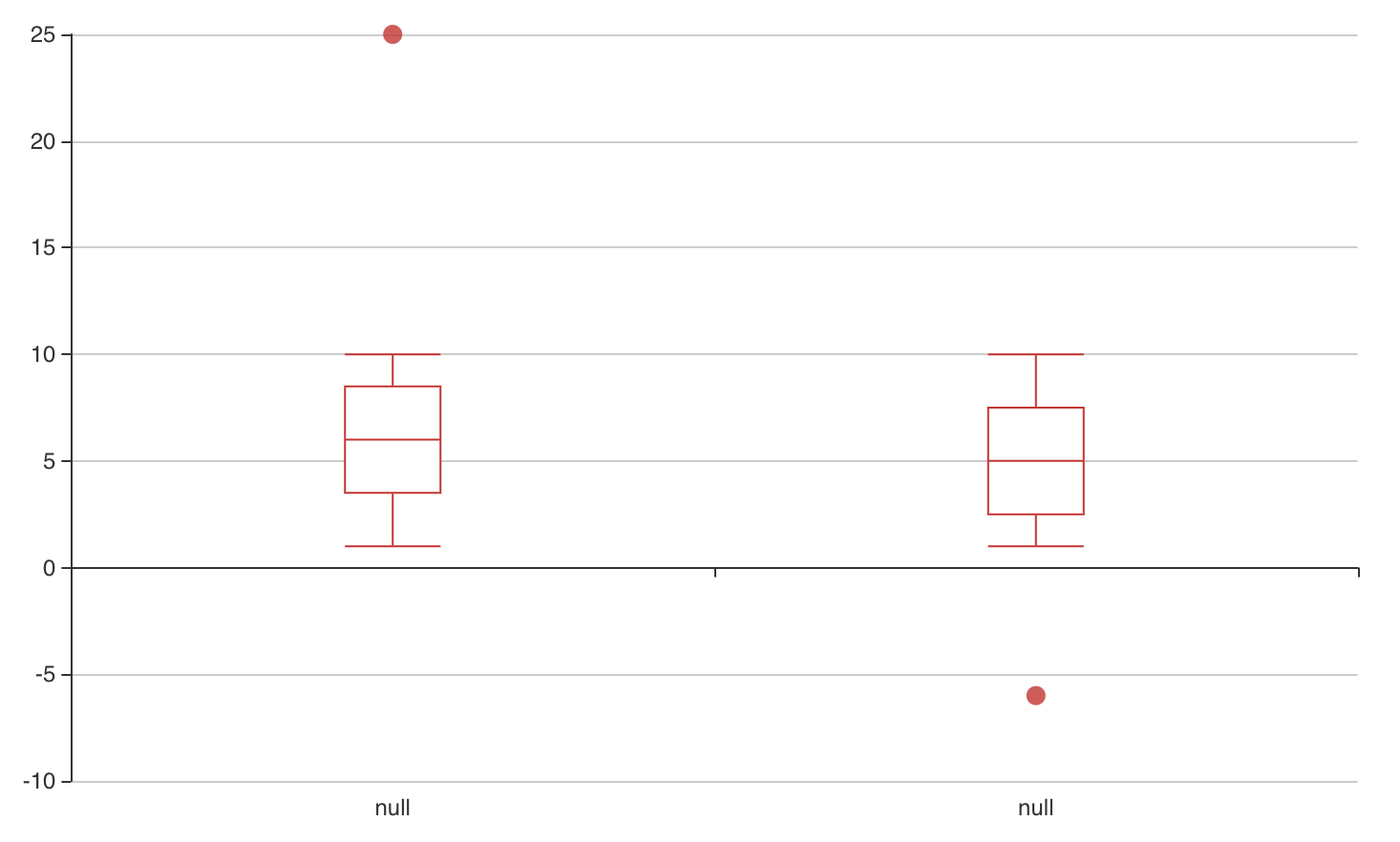/ echarts4r Public

# Using group_by to one-line plot multiple boxplots does not set the x axis labels correctly#219

Closed
opened this issue Oct 22, 2020 · 6 comments
Closed

# Using group_by to one-line plot multiple boxplots does not set the x axis labels correctly #219

opened this issue Oct 22, 2020 · 6 comments
Labels
bug Something isn't working

### lianos commented Oct 22, 2020

 First: thank you for the package, I've only just encountered it, but it looks like it has a lot of promise. It seems like in order to draw multiple box plots on the x-axis w/ out writing `e_boxplot(...)` for every value over x, we need to use `group_by()` So, taking the example from the `e_boxplot()` man page which has data in a wide format, we have: ```library(dplyr) library(echarts4r) df <- data.frame( x = c(1:10, 25), y = c(1:10, -6)) df %>% e_charts() %>% e_boxplot(x, outliers = TRUE) %>% e_boxplot(y, outliers = TRUE)``` If we had multiple categories we wanted to create boxplots for across the x-axis, that can get tedious, so it seems we need to rather convert the data into a long format, and use group_by, like so: ```dfl <- data.frame( var = rep(c("x", "y"), each = nrow(df)), value = c(df\$x, df\$y)) dfl %>% group_by(var) %>% e_charts() %>% e_boxplot(value)``` Just want to clarify that this is the suggested way to do that(?) The issue is that when we plot the data in this way, the labels on the x-axis or the boxplots are all "value" instead of "x" and "y". Perhaps there is a way to use the `name` parameter in the `e_boxplot()` to address this, but not quite sure how if so. Thanks! The text was updated successfully, but these errors were encountered:

### swsoyee commented Oct 23, 2020 • edited

 @lianos I have check the source code, and the labels of x-axis may not be set properly when using `group_by()` function. But you could manually set labels by using `e_x_axis()` at present. Documents are here: https://echarts.apache.org/en/option.html#xAxis.data ```library(dplyr) library(echarts4r) dfl <- data.frame( var = rep(c("x", "y"), each = nrow(df)), value = c(df\$x, df\$y)) dfl %>% group_by(var) %>% e_charts() %>% e_boxplot(value) %>% e_x_axis(data = c("x", "y"))```

### lianos commented Oct 23, 2020 • edited

 I see, thanks for digging around in the codebase! For now, I'd be too nervous to use that approach as I fear I'd be mislabeling the order of the x-axis. I'm not all that familiar with the codebase just yet, but it seems you chased the code logic down to the `.name_it()` call from within the `e_boxplot_()` function? Poking around, here's a naive way for a quick fix to the codebase that looks to be working on my side, if we replace the `.name_it()` call on this line in `add_.R` with the snippet below, it seems to do the trick: ``` if (length(e\$x\$data) > 1 && serie == name) { nm <- .name_it(e, serie, names(e\$x\$data)[i], i) } else { nm <- .name_it(e, serie, name, i) }``` Now my original snippet labels the x axes correctly: ```dfl %>% group_by(var) %>% e_charts() %>% e_boxplot(value)``` However probably won't be so simple when it's done "professionally" :-) Just having some fun, thanks! [note I edited this comment because my first shot at this I posted was very wrong]

### JohnCoene commented Oct 24, 2020

 Thanks, there is definitely an issue here somewhere, I'll take a look.

added a commit that referenced this issue Oct 24, 2020
``` #219 correctly assign names to axis in boxplot when using group_by ```
``` 74a8118 ```

### JohnCoene commented Oct 24, 2020

 Pushed a fix, hope this works. ```df <- data.frame( x = c( rnorm(100), runif(100, -5, 10), rnorm(100, 10, 3) ), grp = c( rep(LETTERS, 100), rep(LETTERS, 100), rep(LETTERS, 100) ) ) df %>% group_by(grp) %>% e_charts() %>% e_boxplot(x)```

### lianos commented Oct 24, 2020 • edited

 Hi John, Thanks for taking a look. This does indeed fix the `group_by()` use case, however the original code that users will find in the Example section of `e_boxplot()` which has data in a wide format and one `e_boxplot()` call per column now has `null` for the x axis label names. For instance: ```df <- data.frame( x = c(1:10, 25), y = c(1:10, -6)) df %>% e_charts() %>% e_boxplot(x, outliers = TRUE) %>% e_boxplot(y, outliers = TRUE)``` Gets you this:### JohnCoene commented Oct 26, 2020

 Thanks for spotting this, just fixed!